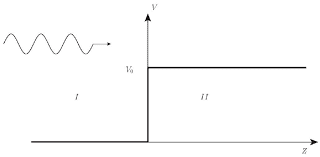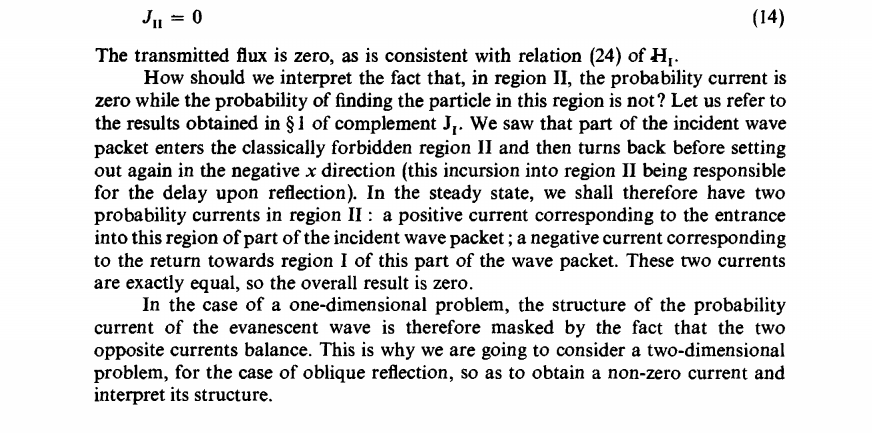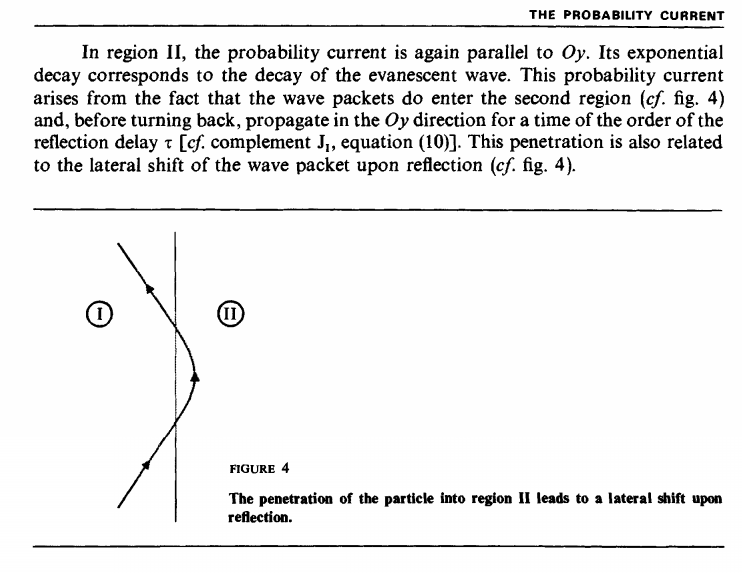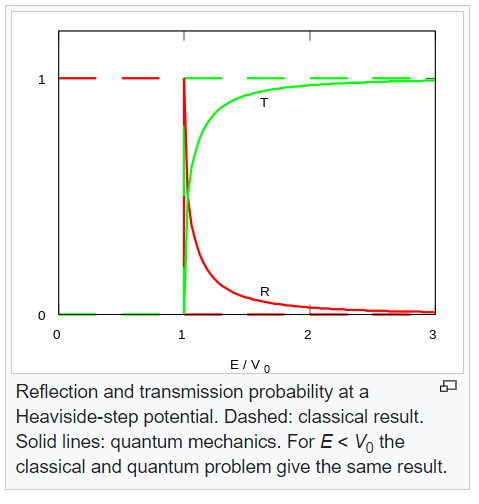# Potential step and tunneling effect

• I
• Maximilian2
In summary, according to the author, when a plane wave with energy less than the potential crosses a potential step, the wave function decays exponentially and the momentum is imaginary.f

#### Maximilian2

TL;DR Summary
How a particle behave when passing through a potential step when its energy is less than the potential itself
We know that thanks to the tunnel effect, in the case of a finite potential step (V) and considering a stationary state, when a plane wave with energy E < V encounter the step the probabability that the wave-particle coming from -∞ (where potential is V=0) will be ≠ 0, in particular the wave function will be exponential decay. We can also calculate the probability flux (J) through the potential step and the result is J=0. In my book i read that taking into account all these results, the interpretation that we can give is that considering many particles, a certain percentage will cross the step and after a definite amount of time it will turns back before setting out in the direction where it came from, this vision allow us to justify why J=0. Here is my question: once (and if) the wave-particle cross the potential step, shouldn't continue its path without turning back? There is a cause that force it to reverse the direction and that can be explain from an "intuitive" point of view?

Hello @Maximilian2 ,##\qquad####\qquad## !

We can also calculate the probability flux (J) through the potential step
Would this be for ##x>a## in the Wikipedia casus ? Or only inside the barier ?

In my book
What book is that ?

I'm referring inside the barier, but in the case of a step potential like in the picture:The book is "Quantum Mechanics" by Claude Choen-Tannoudji, Bernard Diu, and Franck Laloe. (I don't know if it will help but the pages where it's discussed are 65-68, 75-77 and in particular the question i posted is referred to pag. 285) thanks

Ah! Based on the terms 'tunneling'and 'through', I took the barrier example.
For the step function Cohen-Tannoudji chooses appropriate -- careful -- wording.

In theversion I can access on p 282 I find Complement BIII Study of probability current in some special cases / 2. Application to potential barrier problems / b. case where E < V0 :The discussion on 285 is on a two-dimensional case, right ?

But I can't find 'a certain percentage will cross the step' ?

Summary:: How a particle behave when passing through a potential step when its energy is less than the potential itself

In my book i read that taking into account all these results, the interpretation that we can give is that considering many particles, a certain percentage will cross the step and after a definite amount of time it will turns back before setting out in the direction where it came from, this vision allow us to justify why J=0. Here is my question: once (and if) the wave-particle cross the potential step, shouldn't continue its path without turning back? There is a cause that force it to reverse the direction and that can be explain from an "intuitive" point of view?
I think you are referring toAnd that suggests a path through region II for the whole wave packet -- the 'particle'. I find it slightly misleading (but correct). Note that the x-momentum in that region is imaginary !

Re 'shouldn't continue its path without turning back?' No. The wave function decays exponentially and the momentum is imaginary. J = 0. Why should it ?

I am visually oriented so I google 'potential barrier animation' and I like this one and this old one

•Maximilian2
Thanks that helped a lot to clarify. I have just only one more doubt: I don't know if it's correct but when i think about a poential step I naively think about the classic ball that must overcome the slope of an hill, but once it reaches the top (overcome the potential barrier) i can't see what would be the reason that cause the object to turn back; I mean there are no forces nor interactions.
I read in other threads that in the case of a barrier potential we can consider a "sandwich" of different material (https://www.physicsforums.com/threa...rrier-tunneling-and-potential-barrier.334711/), but I'm not sure if it's a correct vision of the problem.

about a potential step I naively think about the classic ball that must overcome the slope of an hill
Not really a step potential, but nevertheless:

Goes to show that a wave is not a tennis ball##\mathstrut \ ##

#### Attachments

•Maximilian2
@vanhees71 : What do you think of fig 4 in post #3 ? Is it exaggerating <x> venturing into x>0 ?

I don't know, how to read this figure (I think you mean #4, right?). The modulus-squared wave function tells us the probability distribution for the position at time ##t##. Why Cohen-Tanoudji draws a trajectory I don't know.

you mean #4, right?
Yes, sorry. I have the feeling <x> stays well within x<0 and am curious about the relationship with the Heisenberg uncertainty (but too lazy/not handy enough to work it out in detail)